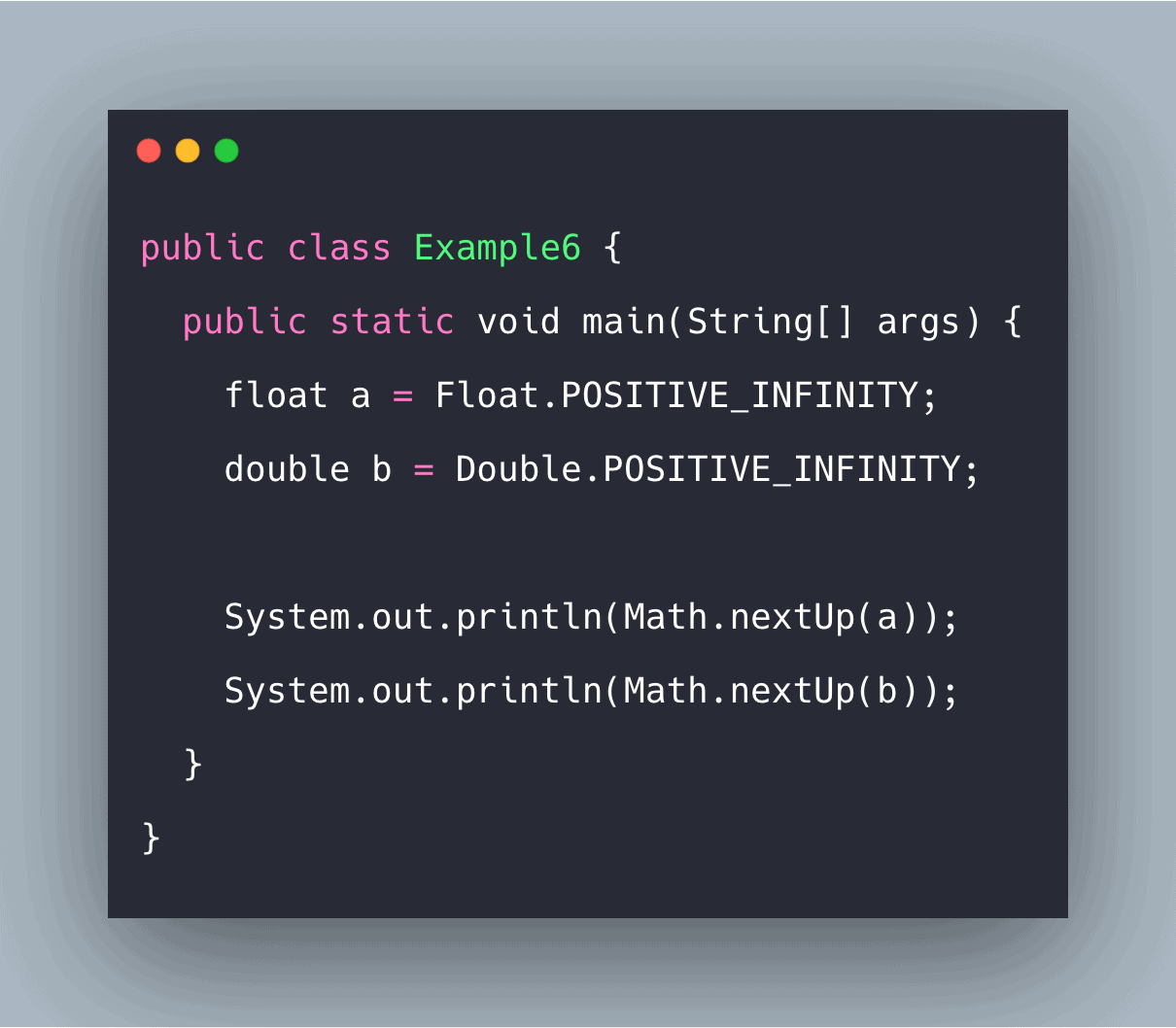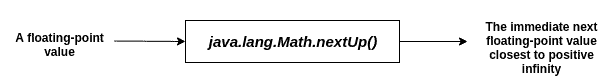# Java Math nextUp(): Complete Guide

0
3Java nextUp() method returns the floating-point value adjacent to the given number in the direction of positive infinity.

## Java Math nextUp()

Java Math nextUp() is a built-in method that is used to find the immediate next(closest to positive infinity) floating-point value that exists after the value is passed as a parameter.

The nextUp() method comes in handy because floating-point numbers are not equidistant and are finite in number. It is to be noted that a nextUp implementation of the equivalent nextAfter implementation may run faster.

### Syntax

```public static float nextUp(float a)
public static double nextUp(double a)
```

### Parameter(s)

A floating-point value (float or double).

### Return Value

The immediate next(closest to positive infinity) floating-point value that exists after the passed value.

See the following figure.#### Note

1. This method is a semantic equivalent of nextAfter(d, Double.POSITIVE_INFINITY) [or nextAfter(f, Float.POSITIVE_INFINITY)].
2. If an argument is NaN, then this method returns NaN.
3. If an argument is zero, then this method returns Double.MIN_VALUE(for double type) or Float.MIN_VALUE(for float type).
4. If an argument is a positive infinity, this method returns positive infinity.

Consider the following examples.

#### Example1.java: The following example demonstrates the use of this method.

```public class Example1{
public static void main(String [] args){
float a = 13.4f;
double b = 13.4;

System.out.println(Math.nextUp(a));
System.out.println(Math.nextUp(b));
}
}
```

#### Output

```Output:
->javac Example1.java
->java Example1
13.400001
13.400000000000002
```

#### Example2.java: The following example demonstrates the use of this method on negative floating-point values.

See the following code.

```public class Example2{
public static void main(String [] args){
float a = -13.4f;
double b = -13.4;

System.out.println(Math.nextUp(a));
System.out.println(Math.nextUp(b));
}
}
```

#### Output

```->javac Example2.java
->java Example2
-13.399999
-13.399999999999999
```

#### Example3.java: The following example demonstrates the relation between nextUp and nextAfter methods.

See the following code.

```public class Example3 {
public static void main(String[] args) {
float a = 104.451f;
double b = 736.147;

System.out.println(Math.nextUp(a));
System.out.println(Math.nextAfter(a, Float.POSITIVE_INFINITY));

System.out.println(Math.nextUp(b));
System.out.println(Math.nextAfter(b, Double.POSITIVE_INFINITY));
}
}
```

#### Output

```->javac Example3.java
->java Example3
104.451004
104.451004
736.1470000000002
736.1470000000002```

#### Example4.java: The following example demonstrates the situation of passing NaN.

See the following code.

```public class Example4 {
public static void main(String[] args) {
float a = Float.NaN;
double b = Double.NaN;

System.out.println(Math.nextUp(a));
System.out.println(Math.nextUp(b));
}
}
```

#### Output

```->javac Example4.java
->java Example4
NaN
NaN
```

#### Example5.java: The following example demonstrates the situation of passing zero.

See the following code.

```public class Example5 {
public static void main(String[] args) {
float a = 0.0f;
double b = 0.0;

System.out.println(Math.nextUp(a));
System.out.println(Float.MIN_VALUE);
System.out.println(Math.nextUp(b));
System.out.println(Double.MIN_VALUE);
}
}
```

#### Output

```->javac Example5.java
->java Example5
1.4E-45
1.4E-45
4.9E-324
4.9E-324```

#### Example6.java: The following example demonstrates the situation of passing positive infinity.

See the following code.

```public class Example6 {
public static void main(String[] args) {
float a = Float.POSITIVE_INFINITY;
double b = Double.POSITIVE_INFINITY;

System.out.println(Math.nextUp(a));
System.out.println(Math.nextUp(b));
}
}
```

#### Output

```->javac Example6.java
->java Example6
Infinity
Infinity
```